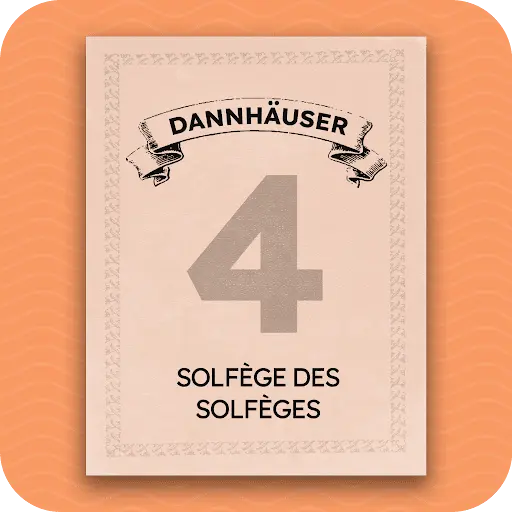Mastering Solfège (Part 4)

This course contains continues from Part 3 of the Mastering Solfège series. Sing exercises from A. Dannhäuser, Solfège des Solfèges, Book 1 (1891). This course will introduce you to the chromatic notes - sharps and flats. These are di (do-#), ri (re-#), fi (fa-#), si (sol-#), li (la-#) and ra (re-b), ma (mi-b), lu (la-b) and ta (ti-b).

Course contents

Singing Fi (Fa-#)Introduction to Fi (Fa-#)Melody using Fi - 1Melody using Fi - 2Melody using Fi - 3

Other Sharp NotesIntroduction to Di (Do-#)Introduction to Si (Sol-#)Introduction to Ri (Re-#)Introduction to Li (La-#)

Flat NotesIntroduction to Ta (Ti-b)Introduction to Ma (Mi-b)Introduction to Lu (La-b)Introduction to Ra (Re-b)

Practice with Sharps and FlatsExercise 1Exercise 2Exercise 3Exercise 4Exercise 5